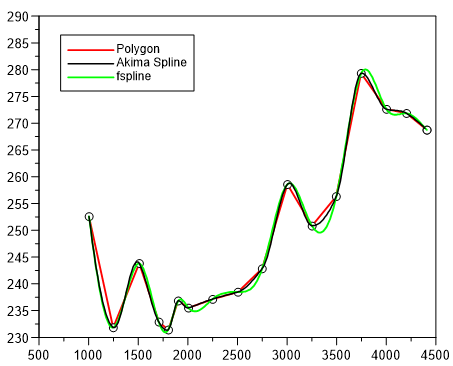# akimaspline¶

akimaspline computes an akima spline for a given x- and y-vector.

`rmXY = akimaspline(rvX, rvY, p, flag)`

Return Value

The return value rmXY is a real matrix with two columns. The first column contains the x-coordinates of the spline and the second column contains the y-coordinates of the spline. In case of an error rmXY has only one element with an error number:

 1 not enough memory 2 x-coordinates are not strictly increasing 3 less than 3 datapoints specified.

Parameters

`rvX`

rvX is a real vector with at least 3 elements in increasing order. The values must be strictly increasing.

`rvY`

rvY is a real vector with at least 3 elememts.

`p`

The meaning of p depends on the value of the parameter flag

`flag`

flag can be one of the following values:

Value Meaning
1 p is the number of x-, y-coordinates calculated.
2 p is the number of x-, y-coordinates calculated. The original x-coordinates are added to the total.
3 p is a strictly increasing vector of x-coordinates.

Example```x = [1,3,5,8]
y = [0,5,8,2]
h = plot(x,y)
xy = akimaspline(x,y,50,2)
plot(xy[;1],xy[;2],h)
xy = fspline(x,y,0,50)
plot(xy[;1],xy[;2],h)
```